# Describe the shape and orientation (direction of positive motion) of the following parametric curve: X =...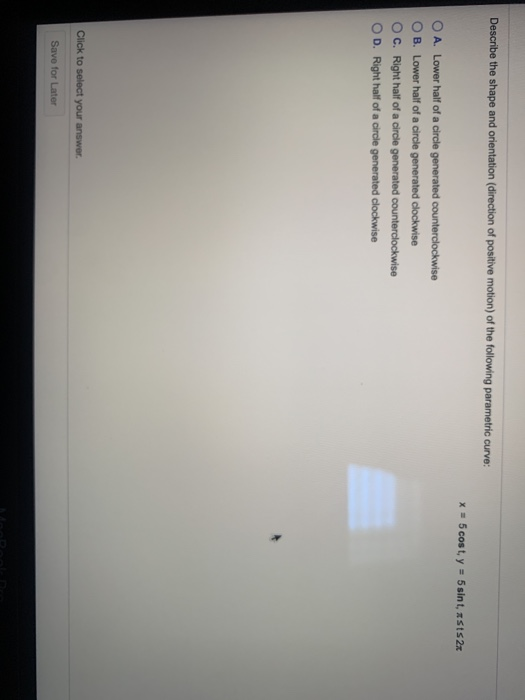Describe the shape and orientation (direction of positive motion) of the following parametric curve: X = 5 cost, y = 5 sint, SS2x O A. Lower half of a circle generated counterclockwise B. Lower half of a circle generated clockwise C. Right half of a circle generated counterclockwise OD. Right half of a circle generated clockwise Click to select your answer. Save for Later

Option 'A' is correct choice.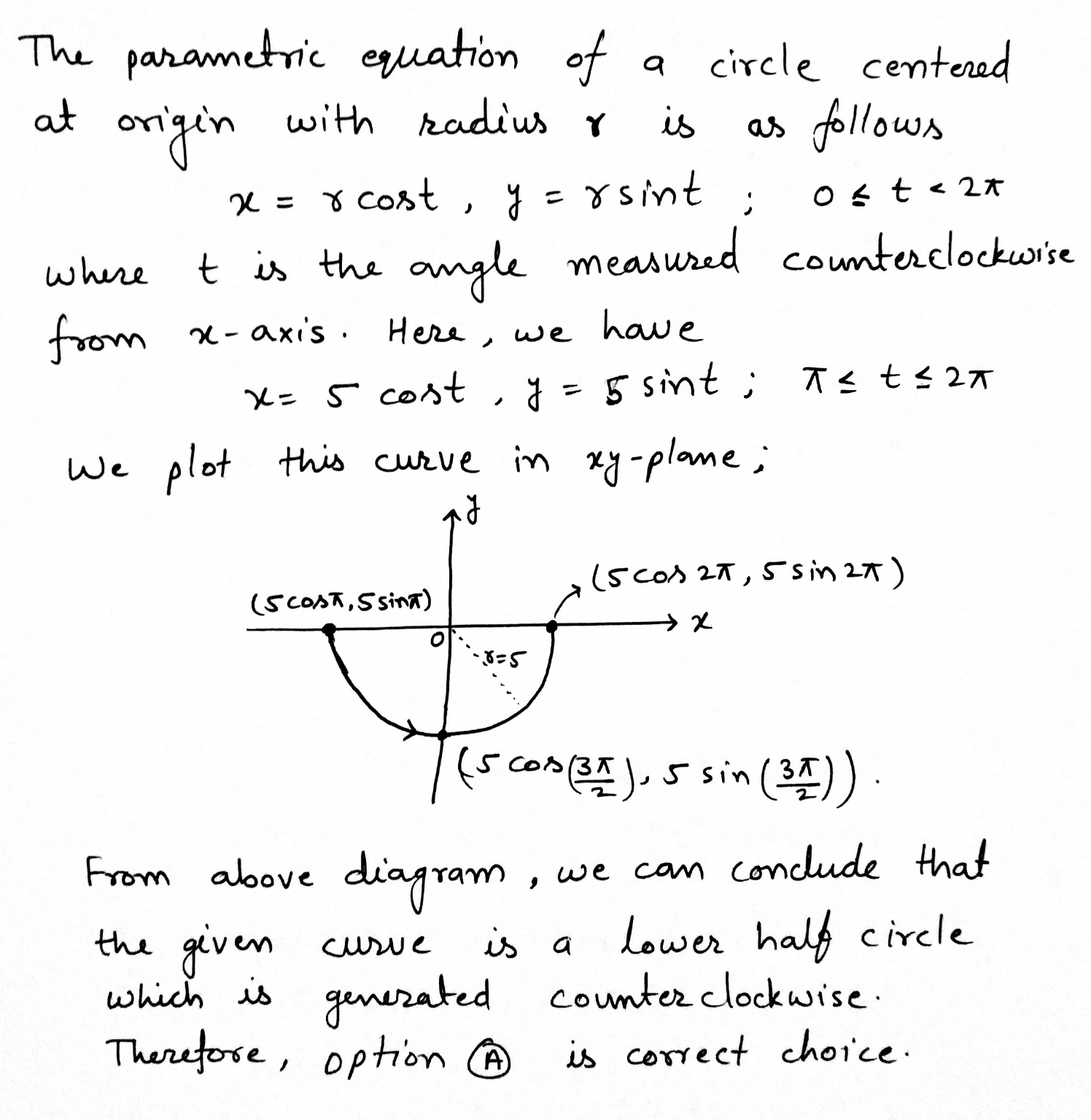#### Earn Coin

Coins can be redeemed for fabulous gifts.

Similar Homework Help Questions
• ### Give parametric equations that describe a full circle of radius​ R, centered at the origin with...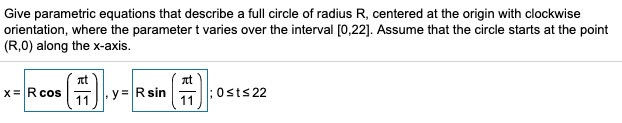Give parametric equations that describe a full circle of radius​ R, centered at the origin with clockwise​ orientation, where the parameter t varies over the interval ​[0,22​]. Assume that the circle starts at the point​ (R,0) along the​ x-axis. Consider the following parametric​ equations, x=−t+7​, y=−3t−3​; minus−5less than or equals≤tless than or equals≤5. Complete parts​ (a) through​ (d) below. Consider the following parametric equation. a.Eliminate the parameter to obtain an equation in x and y. b.Describe the curve and indicate...

• ### The position of an object in circular motion is modeled by the given parametric equations. Describe...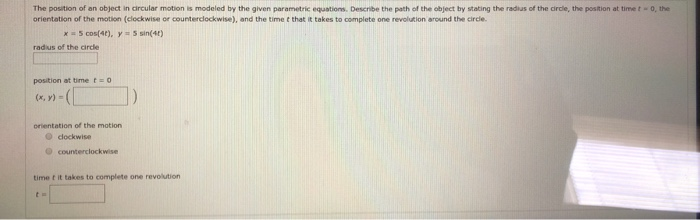The position of an object in circular motion is modeled by the given parametric equations. Describe the path of the object by stating the radius of the circle, the position at time to the orientation of the motion (clockwise or counterclockwise), and the time that it takes to complete one revolution around the circle. x = 5 cos(4), y = sin(40) radius of the circle position at time to (x, y) = orientation of the motion dockwise counterclockwise time it...

• ### Graph the curve whose parametric equations are given and show its orientation. Find the rectangular equation...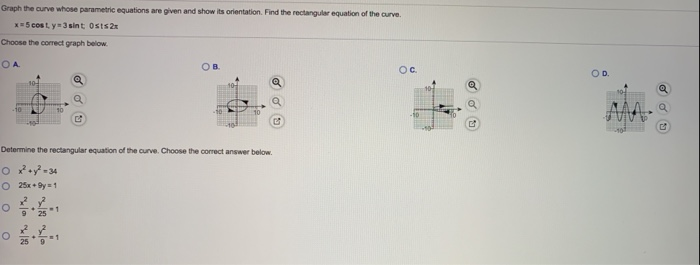Graph the curve whose parametric equations are given and show its orientation. Find the rectangular equation of the curve. x=5 cost. y 3 sint Osts 2x Choose the correct graph below ОА OB Ос OD Q Determine the rectangular equation of the curve. Choose the correct answer below. Ox+y? - 34 O 25x + 9 = 1

• ### Graph the curve whose parametric equations are given and show its orientation. Find the rectangular equation...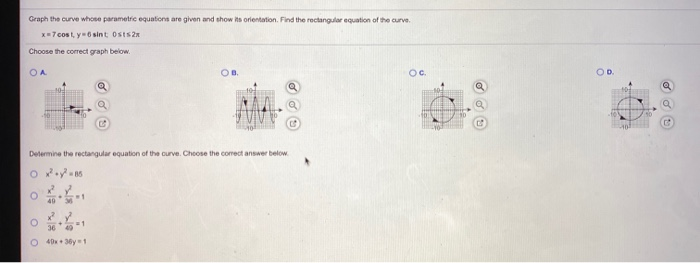Graph the curve whose parametric equations are given and show its orientation. Find the rectangular equation of the curve x=7 cost. y sint Osts 2x Choose the correct graph below. OA OB Ос. OD @ Determine the rectangular equation of the curve. Choose the correct answer below. Oxy7:16 40 O o 36 40 1 0 40x3y1

• ### 12.1.23 Consider the following parametric equations. a. Eliminate the parameter to obtain an equation in x...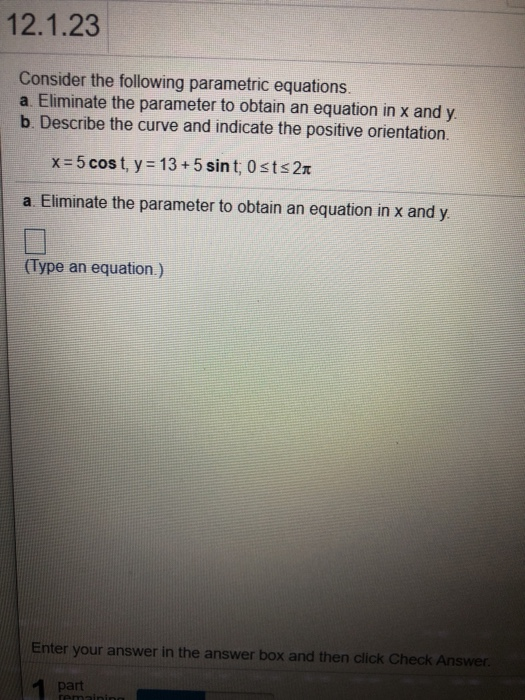12.1.23 Consider the following parametric equations. a. Eliminate the parameter to obtain an equation in x and y b. Describe the curve and indicate the positive orientation. x=5 cost, y = 13 + 5 sint; Osts 21 a. Eliminate the parameter to obtain an equation in x and y. (Type an equation.) Enter your answer in the answer box and then click Check Answer. part remini

• ### Find parametric equations (not unique) for the following circle and give an interval for the parameter....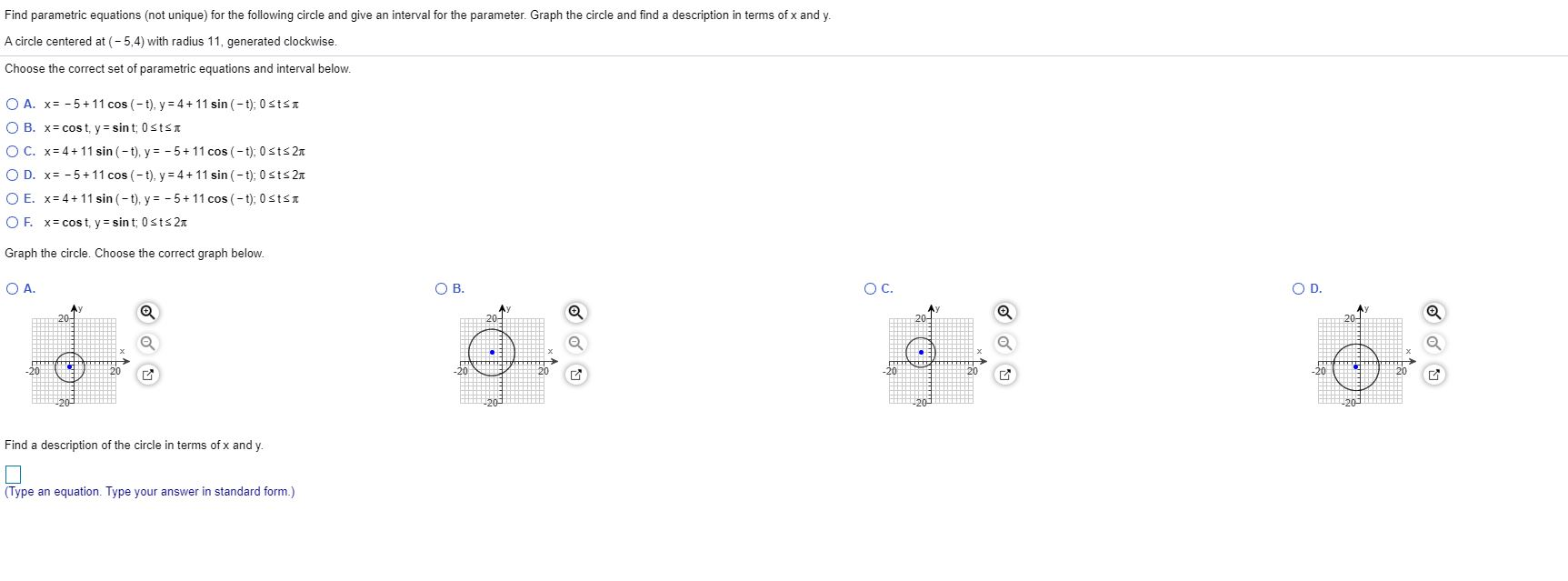Find parametric equations (not unique) for the following circle and give an interval for the parameter. Graph the circle and find a description in terms of x and y. A circle centered at (-5,4) with radius 11, generated clockwise. Choose the correct set of parametric equations and interval below. O A. x= -5+11 cos(-t), y = 4 + 11 sin(-t): 0 SISI OB. x= cost, y = sint: Ostst OC. x= 4 + 11 sin(-t), y = -5 + 11...

• ### Select the first set of parametric equations, x = a cos(bt), y = c sin(dt). (a)...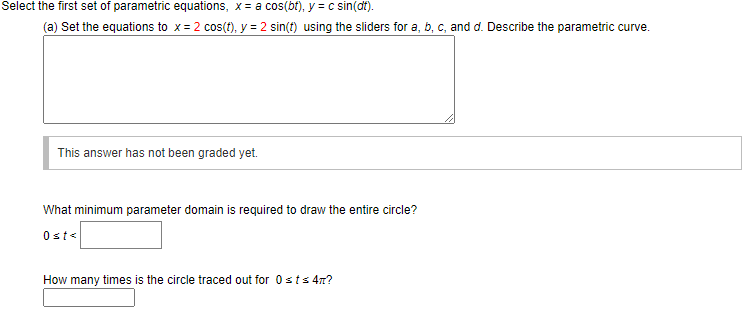Select the first set of parametric equations, x = a cos(bt), y = c sin(dt). (a) Set the equations to x = 2 cos(t), y = 2 sin(t) using the sliders for a, b, c, and d. Describe the parametric curve. This answer has not been graded yet. What minimum parameter domain is required to draw the entire circle? Osts How many times is the circle traced out for Osts 4? Click the Animate button and observe the relationship between...

• ### Given parametric equations and parameter intervals for the motion of a particle in the xy-plane below,...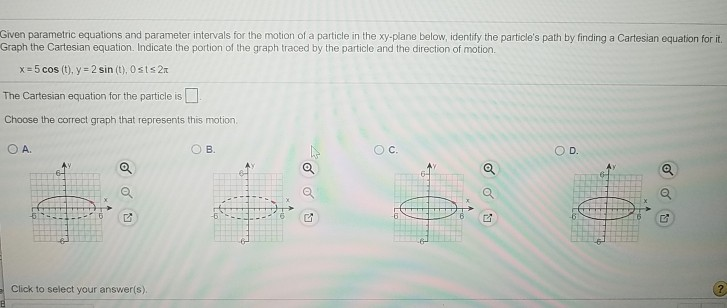Given parametric equations and parameter intervals for the motion of a particle in the xy-plane below, identify the particle's path by finding a Cartesian equation for it Graph the Cartesian equation. Indicate the portion of the graph traced by the particle and the direction of motion. x=5 cos (t), y = 2 sin(t), Osts 2t The Cartesian equation for the particle is Choose the correct graph that represents this motion, OA ОВ. OC OD Q 2 Click to select your...

• ### Verify that the line integral and the surface integral of Stokes Theorem are equal far the following vector field, surface S, and closed curve C. Assume that C has counterlockwise orientation and S h...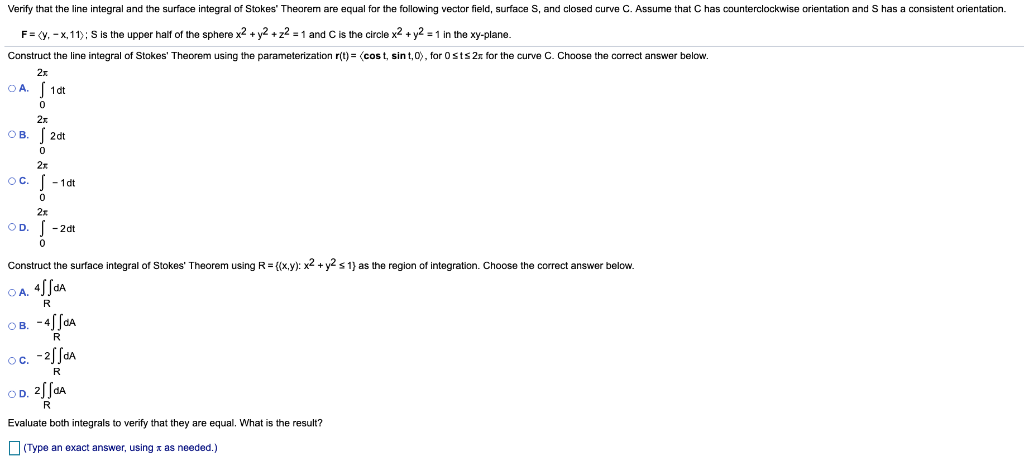Verify that the line integral and the surface integral of Stokes Theorem are equal far the following vector field, surface S, and closed curve C. Assume that C has counterlockwise orientation and S has a consistentorientation F = 〈y,-x, 11), s is the upper half of the sphere x2 + y2 +22-1 and C is the circle x2 + y2-1 in the xy-plane Construct the line integral of Stokes' Theorem using the parameterization r(t)= 〈cost, sint, O. for 0 sts2r...

• ### please help 13) Graph the parametric curve for all real values of x, and indicate the...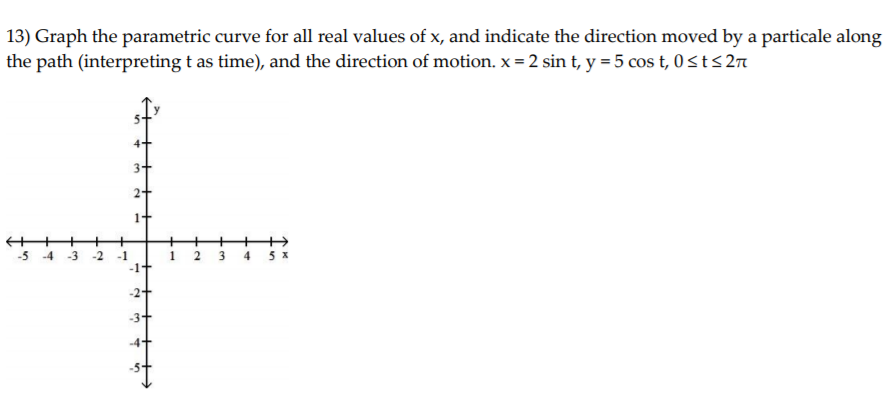please help 13) Graph the parametric curve for all real values of x, and indicate the direction moved by a particale along the path (interpreting t as time), and the direction of motion. x = 2 sin t, y = 5 cos t, Osts 27 2+ 1+ + -5 -4 -3 -2 1 2 - 1 -1+ 3 4 5% 2+ -3+ + Describe the graph of the polar equation sin 20 = 22

Free Homework Help App